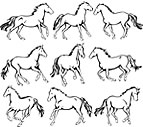×

# arithmetic

1 ENTRIES FOUND:
arithmetic ˈrɪθməˌtɪk/ noun
arithmetic
ˈrɪθməˌtɪk/
noun
Learner's definition of ARITHMETIC
[noncount]
: a branch of mathematics that deals with numbers and their addition, subtraction, multiplication, and division
: the act or process of calculating a number

## — arithmetic